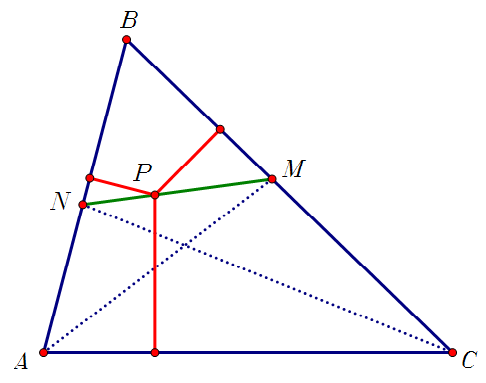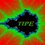# The line through the feet of the bisectors1. In the previous image $AM$ and $CN$ are bisectors and $P$ lies on the line segment $MN$. Prove that the distance from $P$ to line $AC$ is equal to the sum of the distances from $P$ to lines $BA$ and $BC$.

2. What happen if $P$ lies on the line $MN$ but outside the triangle?

3. Now draw the circumcircle of triangle $ABC$ and suppose the ray $MN$ intersects the circumcircle at $Q$ ($N$ is between $Q$ and $M$). Prove that $\frac{1}{QA}=\frac{1}{QC}+\frac{1}{QB}$.Note by Jorge Tipe
7 years, 6 months ago

This discussion board is a place to discuss our Daily Challenges and the math and science related to those challenges. Explanations are more than just a solution — they should explain the steps and thinking strategies that you used to obtain the solution. Comments should further the discussion of math and science.

When posting on Brilliant:

• Use the emojis to react to an explanation, whether you're congratulating a job well done , or just really confused .
• Ask specific questions about the challenge or the steps in somebody's explanation. Well-posed questions can add a lot to the discussion, but posting "I don't understand!" doesn't help anyone.
• Try to contribute something new to the discussion, whether it is an extension, generalization or other idea related to the challenge.

MarkdownAppears as
*italics* or _italics_ italics
**bold** or __bold__ bold
- bulleted- list
• bulleted
• list
1. numbered2. list
1. numbered
2. list
Note: you must add a full line of space before and after lists for them to show up correctly
paragraph 1paragraph 2

paragraph 1

paragraph 2

[example link](https://brilliant.org)example link
> This is a quote
This is a quote
    # I indented these lines
# 4 spaces, and now they show
# up as a code block.

print "hello world"
# I indented these lines
# 4 spaces, and now they show
# up as a code block.

print "hello world"
MathAppears as
Remember to wrap math in $$ ... $$ or $ ... $ to ensure proper formatting.
2 \times 3 $2 \times 3$
2^{34} $2^{34}$
a_{i-1} $a_{i-1}$
\frac{2}{3} $\frac{2}{3}$
\sqrt{2} $\sqrt{2}$
\sum_{i=1}^3 $\sum_{i=1}^3$
\sin \theta $\sin \theta$
\boxed{123} $\boxed{123}$

Sort by:

I proved The first 1... and based on that created a problem. Maybe if u likeJorge,,,, you may share this problem. So here's the problem. In an acute angled triangle $ABC$ we have $BC=4$,$AC=5$,$AB=6$. $BM$ and $CN$ are the internal bisectors of $B$ and $C$. $P$ is any point on the segment $MN$. If $[\triangle PMC]=1$ and $[\triangle PNB]=4$ , find $[\triangle BPC]$ . ( it wud be nice if u mention a little credit to me.. :P )

- 7 years, 6 months ago

Reply if you find this problem interesting! :)

- 7 years, 6 months ago

I think there are too many conditions in your problem. Triangle ABC is fixed and also the points M and N, thus if you choose P in line segment MN such that [BPN]=2 then [CPM] is determined and is not equal to 4. Another idea is to calculate [MNBC] and then find [BPC] (I think the answer will be different of yours).

Try this problem (using your notation):

If [BPN]=2, what is the correct value of [CMP]?

- 7 years, 6 months ago

Then cant we fix $P$ and say that suppose there exists a point $P$ on $MN$ such that ........ ?

- 7 years, 6 months ago

However, solution to #1. Let $PX \perp BC$, $PY \perp AC$ and $PZ \perp AB$. We drop $MM_1 \perp BC$, $MZ_1 \perp AB$ and $NN_1 \perp BC$ and $NY_1 \perp AC$. Now we have $\triangle BM_1M \cong \triangle BMZ_1$ (right angle, angle and side equal) and hence $MZ_1=MM_1$. Similarly we have, $NN_1 = NY_1$.

We use similarity in triangles $MPY$ and $MNY_1$.

$\dfrac{PY}{NY_1} = \dfrac{MP}{NM}$ and we get $PY = \dfrac{PM}{NM} \times NY_1$...(i) Similarly we have $PZ = \dfrac{NP}{NM} \times MZ_1$...(ii)

Now, $NN_1 , PX,MM_1$ are 3 parallel lines . So we have $\dfrac{PM}{NM} = \dfrac{M_1X}{M_1N_1}$.... (iii) We join $N, M_1$ and let that intersect $PX$ at $L$.

Using $\triangle NLP \sim NM_1M$ , we have $\dfrac{PL}{MM_1} = \dfrac{NP}{NM}$ and thus $PL = \dfrac{NP}{NM} \times MM_1 = \dfrac{NP}{NM} \times MZ_1$. (as $MM_1 = MZ_1$ ... (iv)

And, similarly using $\triangle M_1XL \sim \triangle M_1N_1N$ , we have

$LX = \dfrac{XM_1}{N_1M_1} \times NN_1 = \dfrac{PM}{NM} \times NY_1$...(v)

We observe that $(iv) + (v)$ is indeed $(i) + (ii)$ and Hence $PY + PZ = PL + LX = PX$

- 7 years, 6 months ago

Is it correct Jorge??

- 7 years, 6 months ago

It seems good, but you misplaced the points, for example you said $MM_1$ perpendicular to $BC$.

- 7 years, 6 months ago